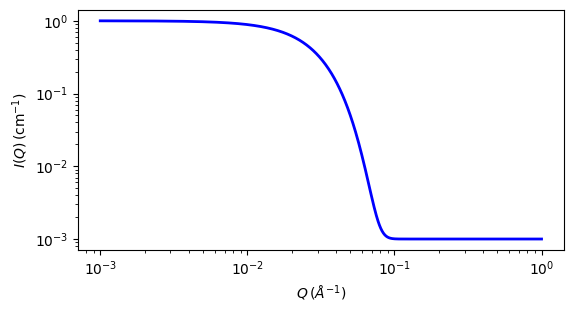# guinier

Parameter

Description

Units

Default value

scale

Scale factor or Volume fraction

None

1

background

Source background

cm-1

0.001

rg

60

The returned value is scaled to units of cm-1 sr-1, absolute scale.

Definition

This model fits the Guinier function

$I(q) = \text{scale} \cdot \exp{\left[ \frac{-Q^2 R_g^2 }{3} \right]} + \text{background}$

to the data directly without any need for linearization (cf. the usual plot of $$\ln I(q)$$ vs $$q^2$$). Note that you may have to restrict the data range to include small q only, where the Guinier approximation actually applies. See also the guinier_porod model.

For 2D data the scattering intensity is calculated in the same way as 1D, where the $$q$$ vector is defined as

$q = \sqrt{q_x^2 + q_y^2}$

In scattering, the radius of gyration $$R_g$$ quantifies the object’s distribution of SLD (not mass density, as in mechanics) from the object’s SLD centre of mass. It is defined by

$R_g^2 = \frac{\sum_i\rho_i\left(r_i-r_0\right)^2}{\sum_i\rho_i}$

where $$r_0$$ denotes the object’s SLD centre of mass and $$\rho_i$$ is the SLD at a point $$i$$.

Notice that $$R_g^2$$ may be negative (since SLD can be negative), which happens when a form factor $$P(Q)$$ is increasing with $$Q$$ rather than decreasing. This can occur for core/shell particles, hollow particles, or for composite particles with domains of different SLDs in a solvent with an SLD close to the average match point. (Alternatively, this might be regarded as there being an internal inter-domain “structure factor” within a single particle which gives rise to a peak in the scattering).

To specify a negative value of $$R_g^2$$ in SasView, simply give $$R_g$$ a negative value ($$R_g^2$$ will be evaluated as $$R_g |R_g|$$). Note that the physical radius of gyration, of the exterior of the particle, will still be large and positive. It is only the apparent size from the small $$Q$$ data that will give a small or negative value of $$R_g^2$$.Fig. 106 1D plot corresponding to the default parameters of the model.

Source

guinier.py

References

1. A Guinier and G Fournet, Small-Angle Scattering of X-Rays, John Wiley & Sons, New York (1955)

Authorship and Verification

• Author: